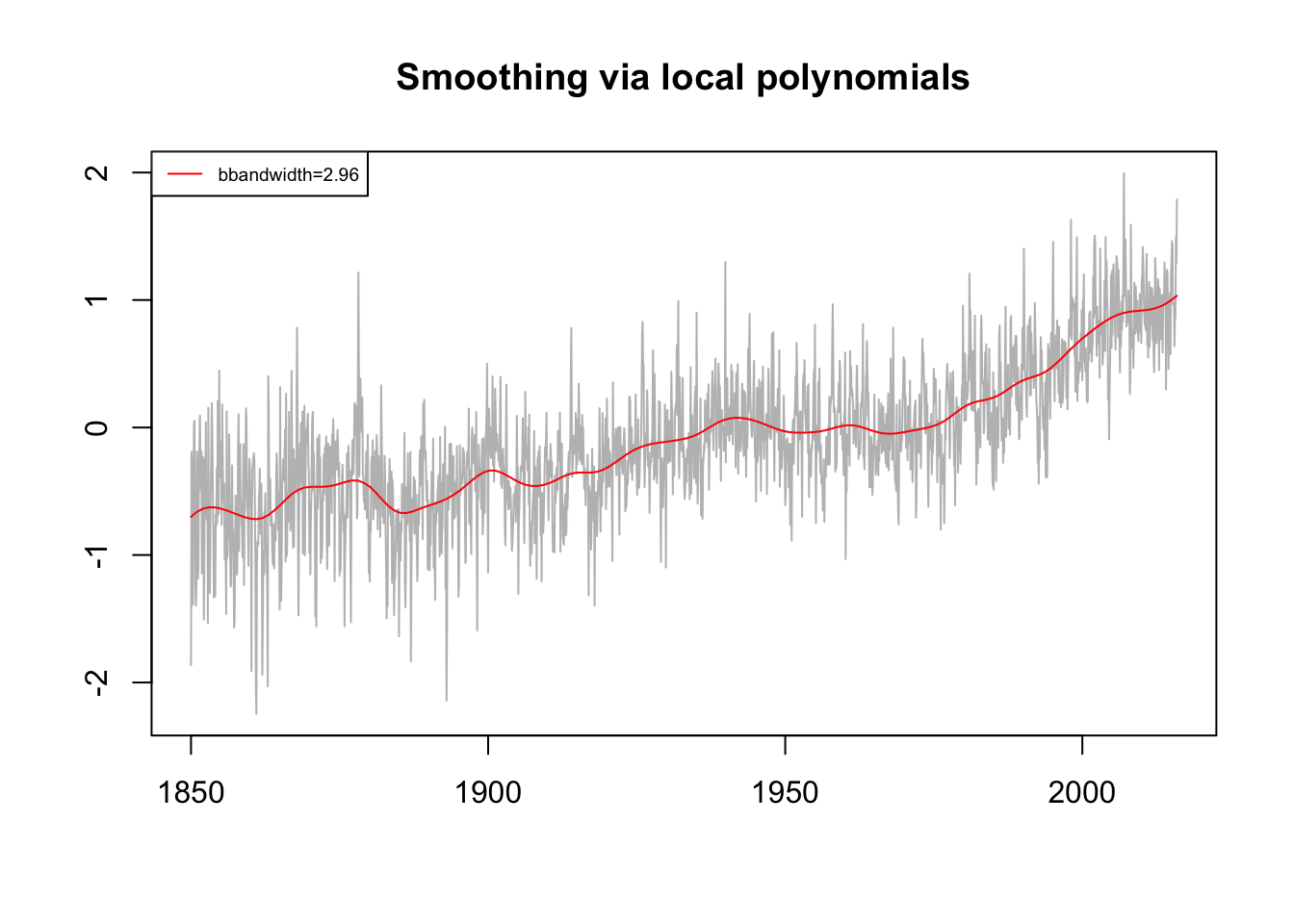A nice implementation of polynomial smoothing in R is provided by the KernSmooth package. The package contains the dpill() function, which helps to select the bandwidth of a local linear Gaussian kernel regression estimate. A small bandwidth means less smoothing, and a large bandwidth means more smoothing

The package includes the locpoly() function, which constructs a polynomial that is fitted to the near-by data points around each data point. The resulting local polynomials create a smoothed version of the original data series.

Both, the dpill() and locpoly() function require to specify number of points (gridsize) for which a local polynomial is constructed.

library(KernSmooth)
library(xts)

dt <- index(temp.global)
y <- coredata(temp.global)
gridsize <- length(dt)/12 # evaluate local polynomial annually (12 months)
# estimate bandwidth of a local linear Gaussian kernel
bandwidth <- dpill(dt, y, gridsize = gridsize)
bandwidth
##  2.956943
# Function estimation using local polynomials
lp <- locpoly(x = dt, y = y,
bandwidth = bandwidth,
gridsize = gridsize)

### PLOTTING ###
plot(dt, y, type = 'l',
col = 'gray', xlab = "", ylab = "",
main = 'Smoothing via local polynomials')

lines(lp, col = 'red', type = 'l')

legend('topleft',
legend = paste0('bbandwidth=', round(bandwidth,2)),
col = 'red',
lty = 1,
cex = 0.6)